Paul's Online Notes
Home / Calculus I / Applications of Integrals / More Volume Problems
Show Mobile Notice Show All Notes Hide All Notes
Mobile Notice
You appear to be on a device with a "narrow" screen width (i.e. you are probably on a mobile phone). Due to the nature of the mathematics on this site it is best views in landscape mode. If your device is not in landscape mode many of the equations will run off the side of your device (should be able to scroll to see them) and some of the menu items will be cut off due to the narrow screen width.

### Section 6.5 : More Volume Problems

In this section we’re going to take a look at some more volume problems. However, the problems we’ll be looking at here will not be solids of revolution as we looked at in the previous two sections. There are many solids out there that cannot be generated as solids of revolution, or at least not easily and so we need to take a look at how to do some of these problems.

Now, having said that these will not be solids of revolutions they will still be worked in pretty much the same manner. For each solid we’ll need to determine the cross-sectional area, either $$A\left( x \right)$$ or $$A\left( y \right)$$, and they use the formulas we used in the previous two sections,

$V = \int_{{\,a}}^{{\,b}}{{A\left( x \right)\,dx}}\hspace{0.75in}V = \int_{{\,c}}^{{\,d}}{{A\left( y \right)\,dy}}$

The “hard” part of these problems will be determining what the cross-sectional area for each solid is. Each problem will be different and so each cross-sectional area will be determined by different means.

Also, before we proceed with any examples we need to acknowledge that the integrals in this section might look a little tricky at first. There are going to be very few numbers in these problems. All of the examples in this section are going to be more general derivation of volume formulas for certain solids. As such we’ll be working with things like circles of radius $$r$$ and we’ll not be giving a specific value of $$r$$ and we’ll have heights of $$h$$ instead of specific heights, etc.

All the letters in the integrals are going to make the integrals look a little tricky, but all you have to remember is that the $$r$$’s and the $$h$$’s are just letters being used to represent a fixed quantity for the problem, i.e. it is a constant. So, when we integrate we only need to worry about the letter in the differential as that is the variable we’re actually integrating with respect to. All other letters in the integral should be thought of as constants. If you have trouble doing that, just think about what you’d do if the $$r$$ was a 2 or the $$h$$ was a 3 for example.

Let’s start with a simple example that we don’t actually need to do an integral that will illustrate how these problems work in general and will get us used to seeing multiple letters in integrals.

Example 1 Find the volume of a cylinder of radius $$r$$ and height $$h$$.
Show Solution

Now, as we mentioned before starting this example we really don’t need to use an integral to find this volume, but it is a good example to illustrate the method we’ll need to use for these types of problems.

We’ll start off with the sketch of the cylinder below.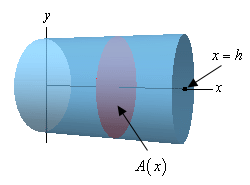We’ll center the cylinder on the $$x$$-axis and the cylinder will start at $$x = 0$$ and end at $$x = h$$ as shown. Note that we’re only choosing this particular set up to get an integral in terms of $$x$$ and to make the limits nice to deal with. There are many other orientations that we could use.

What we need here is to get a formula for the cross-sectional area at any $$x$$. In this case the cross-sectional area is constant and will be a disk of radius $$r$$. Therefore, for any $$x$$ we’ll have the following cross-sectional area,

$A\left( x \right) = \pi {r^2}$

Next the limits for the integral will be $$0 \le x \le h$$ since that is the range of $$x$$ in which the cylinder lives. Here is the integral for the volume,

$V = \int_{0}^{h}{{\pi {r^2}\,dx}} = \pi {r^2}\int_{0}^{h}{{\,dx}} = \left. {\pi {r^2}x} \right|_0^h = \pi {r^2}h$

So, we get the expected formula.

Also, recall we are using $$r$$ to represent the radius of the cylinder. While $$r$$ can clearly take different values it will never change once we start the problem. Cylinders do not change their radius in the middle of a problem and so as we move along the center of the cylinder (i.e. the $$x$$-axis) $$r$$ is a fixed number and won’t change. In other words, it is a constant that will not change as we change the $$x$$. Therefore, because we integrated with respect to $$x$$ the $$r$$ will be a constant as far as the integral is concerned. The $$r$$ can then be pulled out of the integral as shown (although that’s not required, we just did it to make the point). At this point we’re just integrating $$dx$$ and we know how to do that.

When we evaluate the integral remember that the limits are $$x$$ values and so we plug into the $$x$$ and NOT the $$r$$. Again, remember that $$r$$ is just a letter that is being used to represent the radius of the cylinder and, once we start the integration, is assumed to be a fixed constant.

As noted before we started this example if you’re having trouble with the $$r$$ just think of what you’d do if there was a 2 there instead of an $$r$$. In this problem, because we’re integrating with respect to $$x$$, both the 2 and the $$r$$ will behave in the same manner. Note however that you should NEVER actually replace the $$r$$ with a 2 as that WILL lead to a wrong answer. You should just think of what you would do IF the $$r$$ was a 2.

So, to work these problems we’ll first need to get a sketch of the solid with a set of $$x$$ and $$y$$ axes to help us see what’s going on. At the very least we’ll need the sketch to get the limits of the integral, but we will often need it to see just what the cross-sectional area is. Once we have the sketch we’ll need to determine a formula for the cross-sectional area and then do the integral.

Let’s work a couple of more complicated examples. In these examples the main issue is going to be determining what the cross-sectional areas are.

Example 2 Find the volume of a pyramid whose base is a square with sides of length $$L$$ and whose height is $$h$$.
Show Solution

Let’s start off with a sketch of the pyramid. In this case we’ll center the pyramid on the $$y$$-axis and to make the equations easier we are going to position the point of the pyramid at the origin.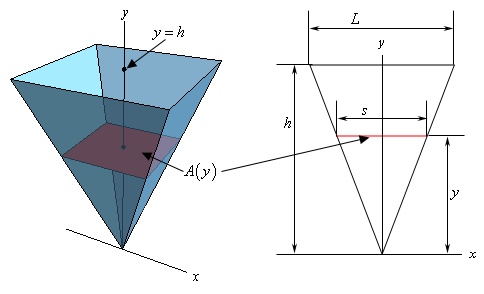Now, as shown here the cross-sectional area will be a function of $$y$$ and it will also be a square with sides of length $$s$$. The area of the square is easy, but we’ll need to get the length of the side in terms of $$y$$. To determine this, consider the figure on the right above. If we look at the pyramid directly from the front we’ll see that we have two similar triangles and we know that the ratio of any two sides of similar triangles must be equal. In other words, we know that,

$\frac{s}{L} = \frac{y}{h}\hspace{0.5in} \Rightarrow \hspace{0.5in}s = \frac{y}{h}L = \frac{L}{h}y$

So, the cross-sectional area is then,

$A\left( y \right) = {s^2} = \frac{{{L^2}}}{{{h^2}}}{y^2}$

The limit for the integral will be $$0 \le y \le h$$ and the volume will be,

$V = \int_{{\,0}}^{{\,h}}{{\frac{{{L^2}}}{{{h^2}}}{y^2}\,dy}} = \frac{{{L^2}}}{{{h^2}}}\int_{{\,0}}^{{\,h}}{{{y^2}\,dy}} = \left. {\frac{{{L^2}}}{{{h^2}}}\left( {\frac{1}{3}{y^3}} \right)} \right|_0^h = \frac{1}{3}{L^2}h$

Again, do not get excited about the $$L$$ and the $$h$$ in the integral. Once we start the problem if we change $$y$$ they will not change and so they are constants as far as the integral is concerned and can get pulled out of the integral. Also, remember that when we evaluate will only plug the limits into the variable we integrated with respect to, $$y$$ in this case.

Before we proceed with some more complicated examples we should once again remind you to not get excited about the other letters in the integrals. If we’re integrating with respect to $$x$$ or $$y$$ then all other letters in the formula that represent fixed quantities (i.e. radius, height, length of a side, etc.) are just constants and can be treated as such when doing the integral.

Now let’s do some more examples.

Example 3 For a sphere of radius $$r$$ find the volume of the cap of height $$h$$.
Show Solution

A sketch is probably best to illustrate what we’re after here.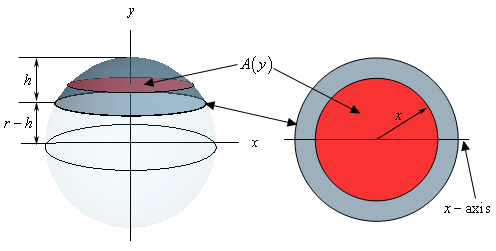We are after the top portion of the sphere and the height of this is portion is $$h$$. In this case we’ll use a cross-sectional area that starts at the bottom of the cap, which is at $$y = r - h$$, and moves up towards the top, which is at $$y = r$$. So, each cross-section will be a disk of radius $$x$$. It is a little easier to see that the radius will be $$x$$ if we look at it from the top as shown in the sketch to the right above. The area of this disk is then,

$\pi {x^2}$

This is a problem however as we need the cross-sectional area in terms of $$y$$. So, what we really need to determine what $${x^2}$$ will be for any given $$y$$ at the cross-section. To get this let’s look at the sphere from the front.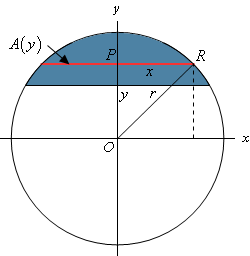In particular look at the triangle POR. Because the point $$R$$ lies on the sphere itself we can see that the length of the hypotenuse of this triangle (the line OR) is $$r$$, the radius of the sphere. The line PR has a length of $$x$$ and the line OP has length $$y$$ so by the Pythagorean Theorem we know,

${x^2} + {y^2} = {r^2}\hspace{0.5in} \Rightarrow \hspace{0.5in}{x^2} = {r^2} - {y^2}$

So, we now know what $${x^2}$$ will be for any given $$y$$ and so the cross-sectional area is,

$A\left( y \right) = \pi \left( {{r^2} - {y^2}} \right)$

As we noted earlier the limits on $$y$$ will be $$r - h \le y \le r$$ and so the volume is,

$V = \int_{{r - h}}^{r}{{\pi \left( {{r^2} - {y^2}} \right)\,dy}} = \pi \left. {\left( {{r^2}y - \frac{1}{3}{y^3}} \right)} \right|_{r - h}^r = \pi \left( {{h^2}r - \frac{1}{3}{h^3}} \right) = \pi {h^2}\left( {r - \frac{1}{3}h} \right)$

In the previous example we again saw an $$r$$ in the integral. However, unlike the previous two examples it was not multiplied times the $$x$$ or the $$y$$ and so could not be pulled out of the integral. In this case it was like we were integrating $$4 - {y^2}$$ and we know how to integrate that. So, in this case we need to treat the $${r^2}$$ like the 4 and so when we integrate that we’ll pick up a $$y$$.

Example 4 Find the volume of a wedge cut out of a cylinder of radius $$r$$ if the angle between the top and bottom of the wedge is $$\frac{\pi }{6}$$.
Show Solution

We should really start off with a sketch of just what we’re looking for here.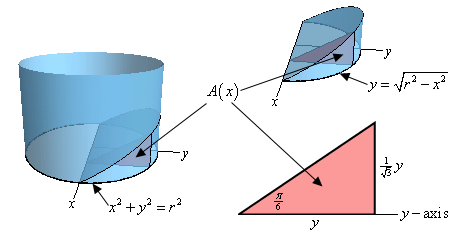On the left we see how the wedge is being cut out of the cylinder. The base of the cylinder is the circle give by $${x^2} + {y^2} = {r^2}$$ and the angle between this circle and the top of the wedge is $$\frac{\pi }{6}$$. The sketch in the upper right position is the actual wedge itself. Given the orientation of the axes here we get the portion of the circle with positive $$y$$ and so we can write the equation of the circle as $$y = \sqrt {{r^2} - {x^2}}$$ since we only need the positive $$y$$ values. Note as well that this is the reason for the way we oriented the axes here. We get positive $$y$$’s and we can write the equation of the circle as a function only of $$x$$’s.

Now, as we can see in the two sketches of the wedge the cross-sectional area will be a right triangle and the area will be a function of $$x$$ as we move from the back of the cylinder, at $$x = - r$$, to the front of the cylinder, at $$x = r$$.

The right angle of the triangle will be on the circle itself while the point on the $$x$$-axis will have an interior angle of $$\frac{\pi }{6}$$. The base of the triangle will have a length of $$y$$ and using a little right triangle trig we see that the height of the rectangle is,

${\mbox{height}} = y\tan \left( {\frac{\pi }{6}} \right) = \frac{1}{{\sqrt 3 }}y$

So, we now know the base and height of our triangle, in terms of $$y$$, and we have an equation for $$y$$ in terms of $$x$$ and so we can see that the area of the triangle, i.e. the cross‑sectional area is,

$A\left( x \right) = \frac{1}{2}\left( y \right)\left( {\frac{1}{{\sqrt 3 }}y} \right) = \frac{1}{2}\sqrt {{r^2} - {x^2}} \left( {\frac{1}{{\sqrt 3 }}\sqrt {{r^2} - {x^2}} } \right) = \frac{1}{{2\sqrt 3 }}\left( {{r^2} - {x^2}} \right)$

The limits on $$x$$ are $$- r \le x \le r$$ and so the volume is then,

$V = \int_{{ - r}}^{r}{{\frac{1}{{2\sqrt 3 }}\left( {{r^2} - {x^2}} \right)}}\,dx = \left. {\frac{1}{{2\sqrt 3 }}\left( {{r^2}x - \frac{1}{3}{x^3}} \right)} \right|_{ - r}^r = \frac{{2{r^3}}}{{3\sqrt 3 }}$

The next example is very similar to the previous one except it can be a little difficult to visualize the solid itself.

Example 5 Find the volume of the solid whose base is a disk of radius $$r$$ and whose cross-sections are equilateral triangles.
Show Solution

Let’s start off with a couple of sketches of this solid. The sketch on the left is from the “front” of the solid and the sketch on the right is more from the top of the solid.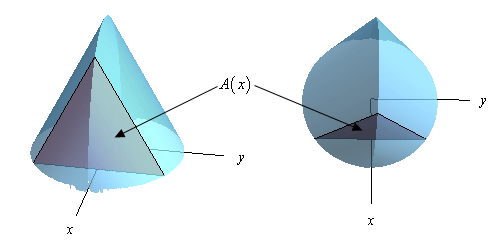The base of this solid is the disk of radius $$r$$ and we move from the back of the disk at $$x = - r$$ to the front of the disk at $$x = r$$ we form equilateral triangles to form the solid. A sample equilateral triangle, which is also the cross-sectional area, is shown above to hopefully make it a little clearer how the solid is formed.

Now, let’s get a formula for the cross-sectional area. Let’s start with the two sketches below.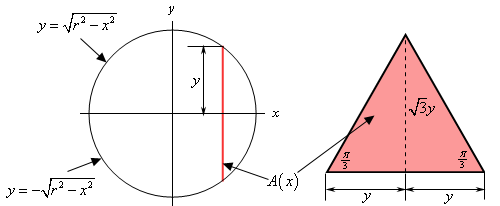In the left hand sketch we are looking at the solid from directly above and notice that we “reoriented” the sketch a little to put the $$x$$ and $$y$$-axis in the “normal” orientation. The solid vertical line in this sketch is the cross-sectional area. From this we can see that the cross-section occurs at a given $$x$$ and the top half will have a length of $$y$$ where the value of $$y$$ will be the $$y$$-coordinate of the point on the circle and so is,

$y = \sqrt {{r^2} - {x^2}}$

Also, because the cross-section is an equilateral triangle that is centered on the $$x$$-axis the bottom half will also have a length of $$y$$. Thus, the base of the cross-section must have a length of 2$$y$$.

The sketch to the right is of one of the cross-sections. As noted above the base of the triangle has a length of 2$$y$$. Also note that because it is an equilateral triangle the angles are all $$\frac{\pi }{3}$$. If we divide the cross-section in two (as shown with the dashed line) we now have two right triangles and using right triangle trig we can see that the length of the dashed line is,

${\mbox{dashed line}} = y\tan \left( {\frac{\pi }{3}} \right) = \sqrt 3 \,y$

Therefore, the height of the cross-section is $$\sqrt 3 \,y$$. Because the cross-section is a triangle we know that that it’s area must then be,

$A\left( x \right) = \frac{1}{2}\left( {2y} \right)\left( {\sqrt 3 \,y} \right) = \frac{1}{2}\left( {2\sqrt {{r^2} - {x^2}} } \right)\left( {\sqrt 3 \,\sqrt {{r^2} - {x^2}} } \right) = \sqrt 3 \left( {{r^2} - {x^2}} \right)$

Note that we used the cross-sectional area in terms of $$x$$ because each of the cross-sections is perpendicular to the $$x$$-axis and this pretty much forces us to integrate with respect to $$x$$.

The volume of the solid is then,

$V\left( x \right) = \int_{{ - \,r}}^{r}{{\sqrt 3 \left( {{r^2} - {x^2}} \right)\,dx}} = \left. {\sqrt 3 \left( {{r^2}x - \frac{1}{3}{x^3}} \right)} \right|_{ - \,r}^r = \frac{4}{{\sqrt 3 }}{r^3}$

The final example we’re going to work here is a little tricky both in seeing how to set it up and in doing the integral.

Example 6 Find the volume of a torus with radii $$r$$ and $$R$$.
Show Solution

First, just what is a torus? A torus is a donut shaped solid that is generated by rotating the circle of radius $$r$$ and centered at ($$R$$, 0) about the $$y$$-axis. This is shown in the sketch to the left below.One of the trickiest parts of this problem is seeing what the cross-sectional area needs to be. There is an obvious one. Most people would probably think of using the circle of radius $$r$$ that we’re rotating about the $$y$$-axis as the cross-section. It is definitely one of the more obvious choices, however setting up an integral using this is not so easy.

So, what we’ll do is use a cross-section as shown in the sketch to the right above. If we cut the torus perpendicular to the $$y$$-axis we’ll get a cross-section of a ring and finding the area of that shouldn’t be too bad. To do that let’s take a look at the two sketches below.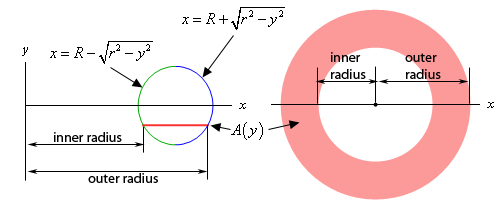The sketch to the left is a sketch of the full cross-section. The sketch to the right is more important however. This is a sketch of the circle that we are rotating about the $$y$$-axis. Included is a line representing where the cross-sectional area would be in the torus.

Notice that the inner radius will always be the left portion of the circle and the outer radius will always be the right portion of the circle. Now, we know that the equation of this is,

${\left( {x - R} \right)^2} + {y^2} = {r^2}$

and so if we solve for $$x$$ we can get the equations for the left and right sides as shown above in the sketch. This however means that we also now have equations for the inner and outer radii.

${\mbox{inner radius}}:x = R - \sqrt {{r^2} - {y^2}} \hspace{0.5in}\hspace{0.25in}{\mbox{outer radius}}:x = R + \sqrt {{r^2} - {y^2}}$

The cross-sectional area is then,

\begin{align*}A\left( y \right) & = \pi {\left( {{\mbox{outer radius}}} \right)^2} - \pi {\left( {{\mbox{inner radius}}} \right)^2}\\ & = \pi \left[ {{{\left( {R + \sqrt {{r^2} - {y^2}} } \right)}^2} - {{\left( {R - \sqrt {{r^2} - {y^2}} } \right)}^2}} \right]\\ & = \pi \left[ {{R^2} + 2R\sqrt {{r^2} - {y^2}} + {r^2} - {y^2} - \left( {{R^2} - 2R\sqrt {{r^2} - {y^2}} + {r^2} - {y^2}} \right)} \right]\\ & = 4\pi R\sqrt {{r^2} - {y^2}} \end{align*}

Next, the lowest cross-section will occur at $$y = - r$$ and the highest cross-section will occur at $$y = r$$ and so the limits for the integral will be $$- r \le y \le r$$.

The integral giving the volume is then,

$V = \int_{{ - r}}^{r}{{4\pi R\sqrt {{r^2} - {y^2}} \,dy}} = 2\int_{0}^{r}{{4\pi R\sqrt {{r^2} - {y^2}} \,dy}} = 8\pi R\int_{0}^{r}{{\sqrt {{r^2} - {y^2}} \,dy}}$

Note that we used the fact that because the integrand is an even function and we’re integrating over $$\left[ { - r,r} \right]$$ we could change the lower limit to zero and double the value of the integral. We saw this fact back in the Computing Definite Integrals section.

We’ve now reached the second really tricky part of this example. With the knowledge that we’ve currently got at this point this integral is not possible to do. It requires something called a trig substitution and that is a topic for Calculus II. Luckily enough for us, and this is the tricky part, in this case we can actually determine the integral’s value using what we know about integrals.

Just for a second let’s think about a different problem. Let’s suppose we wanted to use an integral to determine the area under the portion of the circle of radius $$r$$ and centered at the origin that is in the first quadrant. There are a couple of ways to do this, but to match what we’re doing here let’s do the following.

We know that the equation of the circle is $${x^2} + {y^2} = {r^2}$$ and if we solve for $$x$$ the equation of the circle in the first (and fourth for that matter) quadrant is,

$x = \sqrt {{r^2} - {y^2}}$

If we want an integral for the area in the first quadrant we can think of this area as the region between the curve $$x = \sqrt {{r^2} - {y^2}}$$ and the $$y$$-axis for $$0 \le y \le r$$ and this is,

$A = \int_{0}^{r}{{\sqrt {{r^2} - {y^2}} \,dy}}$

In other words, this integral represents one quarter of the area of a circle of radius $$r$$ and from basic geometric formulas we now know that this integral must have the value,

$A = \int_{0}^{r}{{\sqrt {{r^2} - {y^2}} \,dy}} = \frac{1}{4}\pi {r^2}$

So, putting all this together the volume of the torus is then,

$V = 8R\pi \int_{0}^{r}{{\sqrt {{r^2} - {y^2}} \,dy}} = 8\pi R\left( {\frac{1}{4}\pi {r^2}} \right) = 2R{\pi ^2}{r^2}$